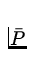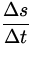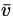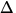# Power

Definition: Power is the time rate of doing work or, the amount of work done per second.

Average Power:== F= F(9)
wheret is the time interval in which the work is done.

Instantaneous Power:

 P = Fv.

Note:

• Power is a scalar.
• SI Units: 1 Watt W ) = 1  Joule/sec = 1  kg m 2s 3
• British Engineering Units: 1 horsepower hp ) = 746  W .